You are currently offline. Some features of the site may not work correctly.

# Kőnig's theorem (graph theory)

Known as: König, König's theorem (graph theory), Koenig theorem (graph theory)
In the mathematical area of graph theory, König's theorem, proved by Dénes Kőnig (), describes an equivalence between the maximum matching problem… Expand
Wikipedia

## Papers overview

Semantic Scholar uses AI to extract papers important to this topic.
2018
2018
• Discret. Math.
• 2018
• Corpus ID: 52067804
Abstract We investigate the relation between Hall’s theorem and Kőnig’s theorem in graphs and hypergraphs. In particular, we… Expand
Is this relevant?
2010
2010
Parameterized complexity is a newly developed sub-area of computational complexity that allows for a more refined analysis of… Expand
•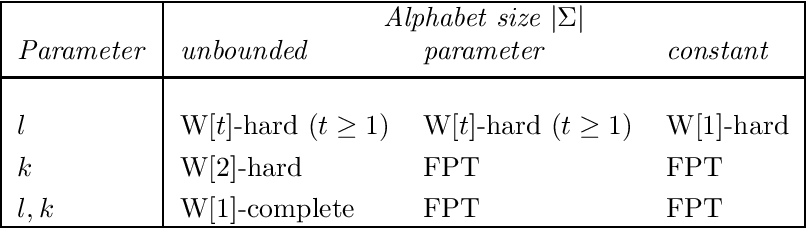•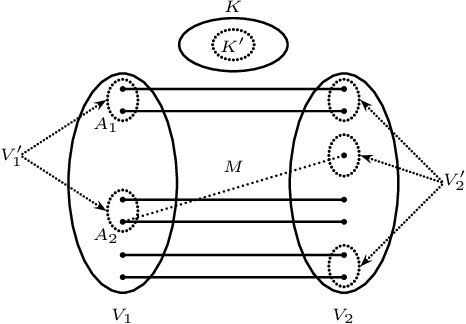•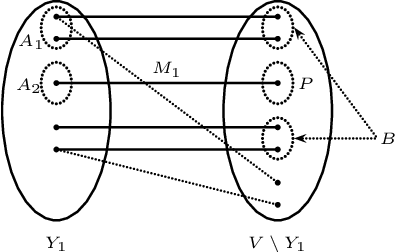•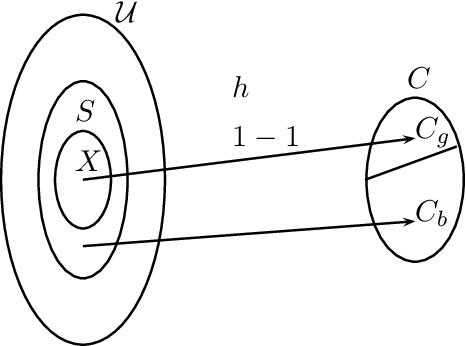•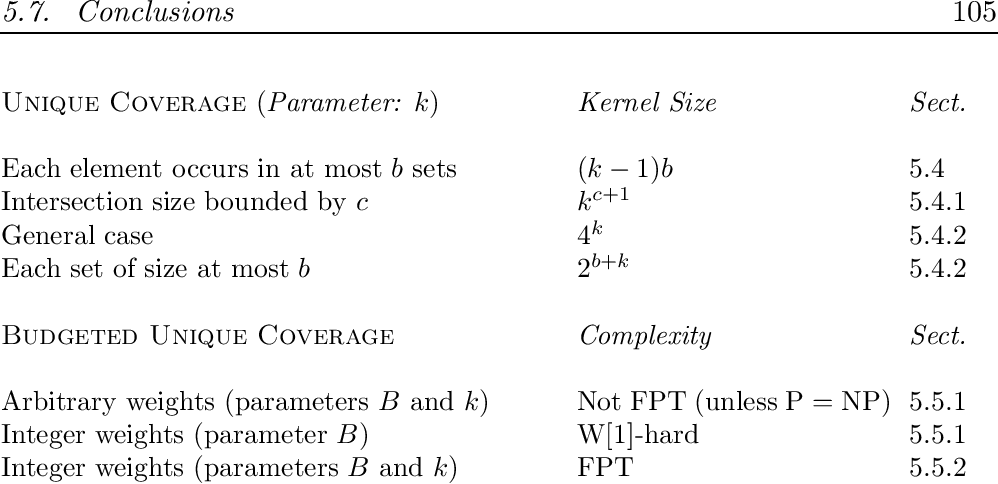Is this relevant?
Review
2008
Review
2008
• 2008
• Corpus ID: 599361
In this column we review the following books. (b) The congruences of a finite lattice: a proof-by picture approach Also by George… Expand
Is this relevant?
2003
2003
• Discret. Math.
• 2003
• Corpus ID: 33687263
The stability number of a graph G, denoted by α(G), is the cardinality of a stable set of maximum size in G. If its stability… Expand
Is this relevant?
2002
2002
• Comb.
• 2002
• Corpus ID: 38277360
Kőnig's theorem states that the covering number and the matching number of a bipartite graph are equal. We prove a generalization… Expand
Is this relevant?